# MOMENT AREA METHODReading time: 1 minute

MOMENT AREA METHOD OF STRUCTURAL ANALYSIS:

Theorem – I

The change in slope between tangents drawn to the elastic curve at any two points A and B is equal to the product of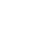and the area of the moment diagrams between those two points (figure 1(a)).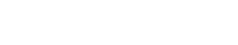Theorem – II

The deviation of any point B relative to a tangent drawn to the elastic curve at any other point A, in a direction perpendicular to the original position of the beam, is equal to the product ofand the moment of area about B of that part of the moment diagram between points A and B (figure 1(b)).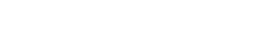Fig.1

This method is useful for finding slope and deflection at a specified position.

Example: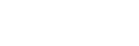(Area of Bending Moment diagram between A and C)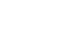(moment of area of the BMD between A and C about C)